https://doi.org/10.5194/acp-18-14465-2018
https://doi.org/10.5194/acp-18-14465-2018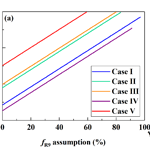# Atmospheric Δ17O(NO3−) reveals nocturnal chemistry dominates nitrate production in Beijing haze

Pengzhen He, Zhouqing Xie, Xiyuan Chi, Xiawei Yu, Shidong Fan, Hui Kang, Cheng Liu, and Haicong Zhan
Abstract

The rapid mass increase of atmospheric nitrate is a critical driving force for the occurrence of fine-particle pollution (referred to as haze hereafter) in Beijing. However, the exact mechanisms for this rapid increase of nitrate mass have not been well constrained from field observations. Here we present the first observations of the oxygen-17 excess of atmospheric nitrate $\left({\mathrm{\Delta }}^{\mathrm{17}}\mathrm{O}\left({\mathrm{NO}}_{\mathrm{3}}^{-}\right)\right)$ collected in Beijing haze to reveal the relative importance of different nitrate formation pathways, and we also present the simultaneously observed ${\mathit{\delta }}^{\mathrm{15}}\mathrm{N}\left({\mathrm{NO}}_{\mathrm{3}}^{-}\right)$. During our sampling period, 12 h averaged mass concentrations of PM2.5 varied from 16 to 323 µg m−3 with a mean of (141±88(1SD)) µg m−3, with nitrate ranging from 0.3 to 106.7 µg m−3. The observed ${\mathrm{\Delta }}^{\mathrm{17}}\mathrm{O}\left({\mathrm{NO}}_{\mathrm{3}}^{-}\right)$ ranged from 27.5 ‰ to 33.9 ‰ with a mean of (30.6±1.8) ‰, while ${\mathit{\delta }}^{\mathrm{15}}\mathrm{N}\left({\mathrm{NO}}_{\mathrm{3}}^{-}\right)$ ranged from −2.5 ‰ to 19.2 ‰ with a mean of (7.4±6.8) ‰. ${\mathrm{\Delta }}^{\mathrm{17}}\mathrm{O}\left({\mathrm{NO}}_{\mathrm{3}}^{-}\right)$-constrained calculations suggest nocturnal pathways (${\mathrm{N}}_{\mathrm{2}}{\mathrm{O}}_{\mathrm{5}}+{\mathrm{H}}_{\mathrm{2}}\mathrm{O}/{\mathrm{Cl}}^{-}$ and NO3+HC) dominated nitrate production during polluted days (PM2.5≥75µg m−3), with a mean possible fraction of 56–97 %. Our results illustrate the potentiality of Δ17O in tracing nitrate formation pathways; future modeling work with the constraint of isotope data reported here may further improve our understanding of the nitrogen cycle during haze.

Share
Dates
1 Introduction

Severe and frequent haze pollution has become a crucial threat for the air quality in the megacity of Beijing and the North China Plain in recent years. The high concentrations of PM2.5 (particulate matter with an aerodynamic diameter equal or less than 2.5 µm) during severe haze, of which the hourly average can reach 1000 µg m−3 (B. Zheng et al., 2015), are harmful to public health by contributing to cardiovascular morbidity and mortality (Cheng et al., 2013; Brook et al., 2010). Nitrate is an important component of PM2.5, accounting for 1–45 % of PM2.5 mass in Beijing and the North China Plain (Wen et al., 2015; B. Zheng et al., 2015; G. Zheng et al., 2015). The main formation pathways of atmospheric nitrate, defined herein as gas-phase HNO3 plus particulate ${\mathrm{NO}}_{\mathrm{3}}^{-}$, in the urban area are summarized in Fig. 1, which includes (i) NO2 oxidation by OH radicals in the gas phase, (ii) heterogeneous uptake of NO2 on wet aerosols, (iii) NO3 radicals reacting with hydrocarbon (HC), and (iv) heterogeneous uptake of N2O5 on wet aerosols and chlorine-containing aerosols. Since OH radicals are mainly present in the daytime, while NO3 radicals and N2O5 are mainly present in the nocturnal atmosphere (Brown and Stutz, 2012), NO2+OH is usually referred to as the daytime nitrate formation pathway, while ${\mathrm{N}}_{\mathrm{2}}{\mathrm{O}}_{\mathrm{5}}+{\mathrm{H}}_{\mathrm{2}}\mathrm{O}/{\mathrm{Cl}}^{-}$ and NO3+HC are referred to as nocturnal formation pathways (Vicars et al., 2013; Sofen et al., 2014). During haze in Beijing, the mixing ratio of daytime OH is modeled to be low (G. Zheng et al., 2015; Rao et al., 2016), while a relatively high mixing ratio of nocturnal N2O5 is observed in several studies (Wang et al., 2017a, b; Li et al., 2018); therefore, nocturnal pathways are suggested to be most responsible for the high concentrations of atmospheric nitrate during haze (Su et al., 2017; Pathak et al., 2009, 2011). In addition, the high PM2.5 concentration and relative humidity during haze in Beijing favor heterogeneous reactions, which renders NO2+H2O a potentially significant pathway for nitrate production (J. Wang et al., 2017; Tong et al., 2015; B. Zheng et al., 2015).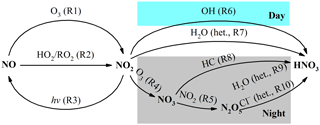Figure 1Simplified schematic of the main nitrate formation pathways in urban air; “het.” refers to heterogeneous reactions on aerosols.

Nitrogen isotopic composition of nitrate (${\mathit{\delta }}^{\mathrm{15}}\mathrm{N}\left({\mathrm{NO}}_{\mathrm{3}}^{-}\right)$, wherein δ15N= (${R}_{\mathrm{sample}}/{R}_{\mathrm{reference}}-\mathrm{1}$), with R representing isotope ratios of 15N∕14N in the sample and the reference atmospheric N2) is useful in tracing the source of its precursor NOX (Xiao et al., 2015; Beyn et al., 2014; Fang et al., 2011; Hastings et al., 2013). Anthropogenic sources of NOX such as coal combustion are generally enriched in δ15N, while natural NOX sources such as soil emissions or lighting typically have a negative or zero δ15N signature (Hoering, 1957; Yu and Elliott, 2017; Felix et al., 2012). Therefore highly positive values of observed ${\mathit{\delta }}^{\mathrm{15}}\mathrm{N}\left({\mathrm{NO}}_{\mathrm{3}}^{-}\right)$ can be considered as an indicator of anthropogenic combustion (Elliott et al., 2009; Fang et al., 2011), although this judgment may be influenced by isotopic exchange between NO and NO2 (Freyer et al., 1993; Walters et al., 2016), isotopic fractionations associated with nitrate formation pathways, and isotopic effects occurring during transport, such as deposition of ${\mathrm{NO}}_{\mathrm{3}}^{-}$ and HNO3 partitioning between the gas and particle phase (Freyer, 1991; Geng et al., 2014). The oxygen-17 excess (Δ17O) of nitrate, defined as ${\mathrm{\Delta }}^{\mathrm{17}}\mathrm{O}={\mathit{\delta }}^{\mathrm{17}}\mathrm{O}-\mathrm{0.52}{\mathit{\delta }}^{\mathrm{18}}\mathrm{O}$, wherein δXO= (${R}_{\mathrm{sample}}/{R}_{\mathrm{reference}}-\mathrm{1}$), with R representing isotope ratios of XO∕16O in the sample and the reference Vienna Standard Mean Ocean Water and X=17 or 18, is particularly useful in reflecting nitrate formation pathways (Michalski et al., 2003). Atmospheric nitrate from nocturnal reaction pathways has higher Δ17O than that from daytime OH oxidation at a given Δ17O(NO2) (Table 1). And once formed, atmospheric ${\mathrm{\Delta }}^{\mathrm{17}}\mathrm{O}\left({\mathrm{NO}}_{\mathrm{3}}^{-}\right)$ cannot be altered by mass-dependent processes such as deposition during transport (Brenninkmeijer et al., 2003). Previous studies have shown the utility of atmospheric ${\mathrm{\Delta }}^{\mathrm{17}}\mathrm{O}\left({\mathrm{NO}}_{\mathrm{3}}^{-}\right)$ in quantifying the relative importance of various nitrate formation pathways (Alexander et al., 2009; Michalski et al., 2003; Patris et al., 2007; Savarino et al., 2013; Vicars et al., 2013). For example, the ${\mathrm{\Delta }}^{\mathrm{17}}\mathrm{O}\left({\mathrm{NO}}_{\mathrm{3}}^{-}\right)$-constrained box modeling work of Michalski et al. (2003) suggests that more than 90 % of atmospheric nitrate is from nocturnal N2O5+H2O pathways in winter in La Jolla, California, which is reflected by the highest ${\mathrm{\Delta }}^{\mathrm{17}}\mathrm{O}\left({\mathrm{NO}}_{\mathrm{3}}^{-}\right)$ values being observed in winter. In another study, Alexander et al. (2009) use observed ${\mathrm{\Delta }}^{\mathrm{17}}\mathrm{O}\left({\mathrm{NO}}_{\mathrm{3}}^{-}\right)$ to constrain a 3-D model and found that the daytime NO2+OH pathway dominates global tropospheric nitrate production, with an annual mean contribution of 76 %.

Table 1Isotope assumptions of different nitrate formation pathways.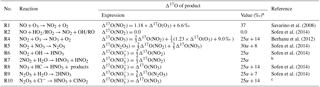a The values are calculated on assumptions that bulk Δ17O(O3)=26 ‰ (Vicars and Savarino, 2014; Ishino et al., 2017) and Δ17O(HO2∕RO2) = 0 ‰. Δ17O(RO2) is equal to 0 ‰ in the troposphere (Morin et al., 2011); in contrast, observations suggest Δ17O(HO2) = 1–2 ‰ (Savarino and Thiemens, 1999). However, the difference in calculated ${\mathrm{\Delta }}^{\mathrm{17}}\mathrm{O}\left({\mathrm{NO}}_{\mathrm{3}}^{-}\right)$ between assuming Δ17O(HO2)= 0 ‰ and Δ17O(HO2)= 2 ‰ is negligible in this study (<0.1 ‰). And the assumption that Δ17O(HO2)= 0 ‰ simplifies calculations and is also consistent with previous studies (Michalski et al., 2003; Alexander et al., 2009; Morin et al., 2008; Kunasek et al., 2008; Sofen et al., 2014). α is the proportion of O3 oxidation in NO2 production rate, calculated by Eq. (3). b Previous studies suggest that in R7 one oxygen atom of ${\mathrm{NO}}_{\mathrm{3}}^{-}$ is from H2O and the other two are from NO2 (Li et al., 2010; Cheung et al., 2000; Goodman et al., 1999), which will result in ${\mathrm{\Delta }}^{\mathrm{17}}\mathrm{O}\left({\mathrm{NO}}_{\mathrm{3}}^{-}\right)$= 23Δ17O(NO2). c R4 and R5 suggest that the central oxygen atom of N2O5 (O2N–O–NO2) is from NO3 radicals (O–NO2), with Δ17O (‰) $=\mathrm{1.23}×{\mathrm{\Delta }}^{\mathrm{17}}\mathrm{O}\left({\mathrm{O}}_{\mathrm{3}}\right)+\mathrm{9.0}$ ‰. R10 is suggested to occur via O2N–O–NO2 (aq.) $\to {\mathrm{NO}}_{\mathrm{2}}^{+}+{\mathrm{NO}}_{\mathrm{3}}^{-}$ and the following ${\mathrm{NO}}_{\mathrm{2}}^{+}+{\mathrm{Cl}}^{-}\to {\mathrm{ClNO}}_{\mathrm{2}}$ (Bertram and Thornton, 2009), so ${\mathrm{\Delta }}^{\mathrm{17}}\mathrm{O}\left({\mathrm{NO}}_{\mathrm{3}}^{-}\right)$$=\mathrm{1}/\mathrm{3}\left(\mathrm{1.23}×{\mathrm{\Delta }}^{\mathrm{17}}\mathrm{O}\left({\mathrm{O}}_{\mathrm{3}}\right)+\mathrm{9.0}$ ‰) $+\mathrm{2}/\mathrm{3}{\mathrm{\Delta }}^{\mathrm{17}}\mathrm{O}\left({\mathrm{NO}}_{\mathrm{2}}\right)={\mathrm{\Delta }}^{\mathrm{17}}\mathrm{O}\left({\mathrm{NO}}_{\mathrm{3}}\right)$.

Until now, however, field observations of atmospheric ${\mathrm{\Delta }}^{\mathrm{17}}\mathrm{O}\left({\mathrm{NO}}_{\mathrm{3}}^{-}\right)$ have not been conducted in north China to constrain the relative importance of different nitrate formation pathways during haze. In this work, we present the first observations of atmospheric ${\mathrm{\Delta }}^{\mathrm{17}}\mathrm{O}\left({\mathrm{NO}}_{\mathrm{3}}^{-}\right)$ during Beijing haze from October 2014 to January 2015, and use this observation to examine the importance of nocturnal formation pathways. We also present the signature of simultaneously observed ${\mathit{\delta }}^{\mathrm{15}}\mathrm{N}\left({\mathrm{NO}}_{\mathrm{3}}^{-}\right)$.

2 Materials and methods

## 2.1 Sampling and atmospheric observations

PM2.5 filter samples were collected at a flow rate of 1.05 m3 min−1 using a high volume air sampler (model TH-1000C II, Tianhong Instruments Co., Ltd, China). The quartz microfiber filter (Whatman Inc., UK) is pre-combusted at 450 C for 4 h before sampling. Our sampling period lasted from October 2014 to January 2015, with the collection interval being 12 h (08:00–20:00 LT or 20:00–08:00 LT) for each sample. Blank control samples were also collected. The blank was sampled identically to the real sample except that the collection interval was 1 min. Due to the fact that gaseous HNO3 is likely to adsorb onto particulate matter already trapped by the filter material (Vicars et al., 2013), the nitrate species collected is likely to include both particulate nitrate and gaseous HNO3, which is referred to as atmospheric nitrate in previous studies (Vicars et al., 2013; Morin et al., 2009; Michalski et al., 2003) and in this study. The sampling site is at the campus of University of the Chinese Academy of Sciences (40.41 N, 116.68 E; ∼20 m high) in suburban Beijing, about 60 km northeast of downtown Beijing (Fig. 2), which is a supersite set up by HOPE-J3A (Haze Observation Project Especially for Jing–Jin–Ji Area), with various observations being reported (Zhang et al., 2017; Xu et al., 2016; Chen et al., 2015; Tong et al., 2015; He et al., 2018). Hourly concentrations of surface PM2.5, CO, SO2, NO2, and O3 were observed at Huairou station (40.33 N, 116.63 E) by Beijing Municipal Environmental Monitoring Center, about 10 km from our sampling site. Meteorological data including relative humidity (RH) and air temperature (T) were measured by an automatic weather station (model MetPak, Gill Instruments Limited, UK). Time used in the present study is local time (LT = UTC +8).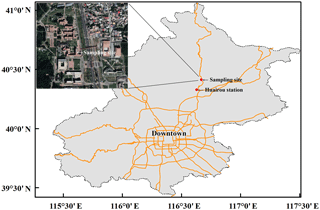Figure 2A brief map of the sampling site in Beijing. The map scale of base map is 1:1 250 000. Huairou station is set up by the Beijing Municipal Environmental Monitoring Center, where hourly PM2.5, SO2, CO, NO2, and O3 were observed.

## 2.2 Measurements of ions and isotopic ratios

Ion concentrations of ${\mathrm{NO}}_{\mathrm{3}}^{-}$ and Cl were measured in Anhui Province Key Laboratory of Polar Environment and Global Change in the University of Science and Technology of China. A detailed description of this method can be found in the literature (Ye et al., 2015). Briefly, ions in the PM2.5 filter sample were extracted with Millipore water (≥18 MΩ) and insoluble substances in the extract were filtered. Then the ion concentrations were analyzed using an ion chromatograph system (model Dionex ICS-2100, Thermo Fisher Scientific Inc., USA). The measured ion concentrations of blank samples were subtracted when determining the ion concentrations of real samples. Typical analytical precision by our method is better than 10 % relative standard deviation (RSD) (Chen et al., 2016).

${\mathit{\delta }}^{\mathrm{15}}\mathrm{N}\left({\mathrm{NO}}_{\mathrm{3}}^{-}\right)$ and ${\mathrm{\Delta }}^{\mathrm{17}}\mathrm{O}\left({\mathrm{NO}}_{\mathrm{3}}^{-}\right)$ were measured with a bacterial denitrifier method (Kaiser et al., 2007) in IsoLab at the University of Washington, USA. Briefly, ions in the filter sample were extracted with Millipore water (≥18 MΩ) and the insoluble substances were filtered. ${\mathrm{NO}}_{\mathrm{3}}^{-}$ in each sample was converted to N2O by the denitrifying bacteria, Pseudomonas aureofaciens. Then N2 and O2, which were decomposed from N2O in a gold tube at 800 C, were separated using a gas chromatograph. The isotopic ratios of each gas were then measured by a Finnigan Delta-Plus Advantage isotope ratio mass spectrometer. Masses of 28 and 29 from N2 were measured to determine δ15N. Masses of 32, 33, and 34 from O2 were measured to determine δ17O and δ18O, and Δ17O was then calculated. We use international nitrate reference materials, USGS34, USGS35, and IAEANO3, for data calibration. The uncertainty (1σ) of δ15N and Δ17O measurements in our method is 0.4 ‰ and 0.2 ‰, respectively, based on replicate analysis of the international reference materials. All the samples including blank samples were measured in triplicate to quantify the uncertainty in each sample. The blank was subtracted for each sample by using an isotopic mass balance on the basis of isotopic ratios and concentrations of the blank. To minimize the blank effect, samples with blank concentrations being >10 % of their concentrations were not analyzed for isotopic ratios. This ruled out 3 of the total 34 samples, all of which are from non-polluted days (NPD, PM2.5< 75 µg m−3). In total, isotopic compositions of 7 samples from NPD and 24 samples from polluted days (PD, PM2.5≥75µg m−3) are reported here.

## 2.3 Estimate of different nitrate formation pathways based on ${\mathrm{\Delta }}^{\mathrm{17}}\mathrm{O}\left({\mathrm{NO}}_{\mathrm{3}}^{-}\right)$

The observed ${\mathrm{\Delta }}^{\mathrm{17}}\mathrm{O}\left({\mathrm{NO}}_{\mathrm{3}}^{-}\right)$ is determined by the relative importance of different nitrate formation pathways and the relative importance of O3 oxidation in NOX cycling as shown in Eq. (1):

$\begin{array}{ll}\mathrm{\Delta }& {}^{\mathrm{17}}\mathrm{O}\left({\mathrm{NO}}_{\mathrm{3}}^{-}\right)={{\mathrm{\Delta }}^{\mathrm{17}}\mathrm{O}}_{\mathrm{R}\mathrm{6}}×{f}_{\mathrm{R}\mathrm{6}}+{{\mathrm{\Delta }}^{\mathrm{17}}\mathrm{O}}_{\mathrm{R}\mathrm{7}}×{f}_{\mathrm{R}\mathrm{7}}+{{\mathrm{\Delta }}^{\mathrm{17}}\mathrm{O}}_{\mathrm{R}\mathrm{8}}\\ \text{(1)}& & ×{f}_{\mathrm{R}\mathrm{8}}+{{\mathrm{\Delta }}^{\mathrm{17}}\mathrm{O}}_{\mathrm{R}\mathrm{9}}×{f}_{\mathrm{R}\mathrm{9}}+{{\mathrm{\Delta }}^{\mathrm{17}}\mathrm{O}}_{\mathrm{R}\mathrm{10}}×{f}_{\mathrm{R}\mathrm{10}},\end{array}$

where Δ17OR6, Δ17OR7, Δ17OR8, Δ17OR9, and Δ17OR10 are, respectively, ${\mathrm{\Delta }}^{\mathrm{17}}\mathrm{O}\left({\mathrm{NO}}_{\mathrm{3}}^{-}\right)$ resulting from NO2+OH, NO2+H2O, NO3+HC, N2O5+H2O, and ${\mathrm{N}}_{\mathrm{2}}{\mathrm{O}}_{\mathrm{5}}+{\mathrm{Cl}}^{-}$ pathways (Table 1); fR6, fR7, fR8, fR9, and fR10 are, respectively, corresponding fractional contributions of the above pathways to nitrate production. Using the Δ17O assumptions for different pathways in Table 1 and the definition ${f}_{\mathrm{R}\mathrm{6}}+{f}_{\mathrm{R}\mathrm{7}}+{f}_{\mathrm{R}\mathrm{8}}+{f}_{\mathrm{R}\mathrm{9}}+{f}_{\mathrm{R}\mathrm{10}}=\mathrm{1}$, Eq. (1) is further expressed as

$\begin{array}{ll}& {\mathrm{\Delta }}^{\mathrm{17}}\mathrm{O}\left({\mathrm{NO}}_{\mathrm{3}}^{-}\right)/\mathrm{‰}=\mathrm{25}\mathit{\alpha }{f}_{\mathrm{R}\mathrm{6}}+\mathrm{25}\mathit{\alpha }{f}_{\mathrm{R}\mathrm{7}}+\left(\mathrm{25}\mathit{\alpha }+\mathrm{14}\right)×{f}_{\mathrm{R}\mathrm{8}}\\ & +\left(\mathrm{25}\mathit{\alpha }+\mathrm{7}\right)×{f}_{\mathrm{R}\mathrm{9}}+\left(\mathrm{25}\mathit{\alpha }+\mathrm{14}\right)×{f}_{\mathrm{R}\mathrm{10}}\\ \text{(2)}& & =\mathrm{25}\mathit{\alpha }+\mathrm{14}×\left({f}_{\mathrm{R}\mathrm{8}}+{f}_{\mathrm{R}\mathrm{10}}\right)+\mathrm{7}{f}_{\mathrm{R}\mathrm{9}},\end{array}$

where α is the proportion of O3 oxidation in NO2 production rate, calculated by Eq. (3):

$\begin{array}{}\text{(3)}& \mathit{\alpha }=\frac{{k}_{\mathrm{R}\mathrm{1}}\left[\mathrm{NO}\right]\left[{\mathrm{O}}_{\mathrm{3}}\right]}{{k}_{\mathrm{R}\mathrm{1}}\left[\mathrm{NO}\right]\left[{\mathrm{O}}_{\mathrm{3}}\right]+{k}_{\mathrm{R}\mathrm{2}\mathrm{a}}\left[\mathrm{NO}\right]\left[{\mathrm{HO}}_{\mathrm{2}}\right]+{k}_{\mathrm{R}\mathrm{2}\mathrm{b}}\left[\mathrm{NO}\right]\left[{\mathrm{RO}}_{\mathrm{2}}\right]}.\end{array}$

In Eq. (3), kR1, kR2a, and kR2b are, respectively, the reaction rate constants listed in Table 2. To evaluate α, we estimated HO2 mixing ratios on the basis of empirical formulas between HO2 and O3 mixing ratios derived from observations in winter (Kanaya et al., 2007); i.e., $\left[{\mathrm{HO}}_{\mathrm{2}}\right]/\left(\mathrm{pmol}\phantom{\rule{0.125em}{0ex}}{\mathrm{mol}}^{-\mathrm{1}}\right)=\mathrm{exp}\left(\mathrm{5.7747}×{\mathrm{10}}^{-\mathrm{2}}×\left[{\mathrm{O}}_{\mathrm{3}}\right]/\left(\mathrm{nmol}\phantom{\rule{0.125em}{0ex}}{\mathrm{mol}}^{-\mathrm{1}}\right)-\mathrm{1.7227}\right)$ during the daytime and $\left[{\mathrm{HO}}_{\mathrm{2}}\right]/\left(\mathrm{pmol}\phantom{\rule{0.125em}{0ex}}{\mathrm{mol}}^{-\mathrm{1}}\right)=\mathrm{exp}\left(\mathrm{7.7234}×{\mathrm{10}}^{-\mathrm{2}}×\left[{\mathrm{O}}_{\mathrm{3}}\right]/\left(\mathrm{nmol}\phantom{\rule{0.125em}{0ex}}{\mathrm{mol}}^{-\mathrm{1}}\right)-\mathrm{1.6363}\right)$ at night. Then the RO2 mixing ratio was calculated as 70 % of HO2 mixing ratios based on previous studies (Liu et al., 2012; Elshorbany et al., 2012; Mihelcic et al., 2003). As the NO mixing ratio was not observed in our study, we estimated NO mixing ratios following the empirical formulas between NOX and CO mixing ratios derived from observations in winter Beijing (Lin et al., 2011); i.e., $\left[\mathrm{NO}\right]/\left(\mathrm{nmol}\phantom{\rule{0.125em}{0ex}}{\mathrm{mol}}^{-\mathrm{1}}\right)=\left(\left[\mathrm{CO}\right]/\left(\mathrm{nmol}\phantom{\rule{0.125em}{0ex}}{\mathrm{mol}}^{-\mathrm{1}}\right)-\mathrm{196}\right)/\mathrm{27.3}-\left[{\mathrm{NO}}_{\mathrm{2}}\right]/\left(\mathrm{nmol}\phantom{\rule{0.125em}{0ex}}{\mathrm{mol}}^{-\mathrm{1}}\right)$ during daytime and $\left[\mathrm{NO}\right]/\left(\mathrm{nmol}\phantom{\rule{0.125em}{0ex}}{\mathrm{mol}}^{-\mathrm{1}}\right)=\left(\left[\mathrm{CO}\right]/\left(\mathrm{nmol}\phantom{\rule{0.125em}{0ex}}{\mathrm{mol}}^{-\mathrm{1}}\right)-\mathrm{105}\right)/\mathrm{30.9}-\left[{\mathrm{NO}}_{\mathrm{2}}\right]/\left(\mathrm{nmol}\phantom{\rule{0.125em}{0ex}}{\mathrm{mol}}^{-\mathrm{1}}\right)$ at night.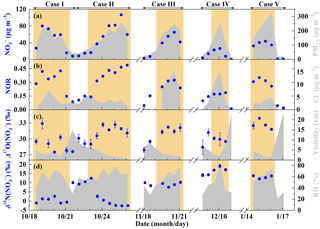Figure 3General characteristics of haze events in Beijing (October 2014–January 2015). (a) Time series of PM2.5 and ${\mathrm{NO}}_{\mathrm{3}}^{-}$ concentrations. (b) Time series of nitrogen oxidation ratio (NOR, which is equal to the ${\mathrm{NO}}_{\mathrm{3}}^{-}$ molar concentration divided by the sum of ${\mathrm{NO}}_{\mathrm{3}}^{-}$ and NO2 molar concentration) and Cl concentrations. (c) Time series of ${\mathrm{\Delta }}^{\mathrm{17}}\mathrm{O}\left({\mathrm{NO}}_{\mathrm{3}}^{-}\right)$ and visibility. (d) Time series of ${\mathit{\delta }}^{\mathrm{15}}\mathrm{N}\left({\mathrm{NO}}_{\mathrm{3}}^{-}\right)$ and relative humidity (RH). The error bars in (c, d) are ±1σ of replicate measurements (n=3) of each sample. The khaki shaded area indicates polluted days (PD, PM2.5≥75µg m−3).

By using Eq. (2), the relative importance of nocturnal formation pathways (${f}_{\mathrm{R}\mathrm{8}}+{f}_{\mathrm{R}\mathrm{9}}+{f}_{\mathrm{R}\mathrm{10}}\right)$ can be written as Eq. (4):

$\begin{array}{}\text{(4)}& {f}_{\mathrm{R}\mathrm{8}}+{f}_{\mathrm{R}\mathrm{9}}+{f}_{\mathrm{R}\mathrm{10}}=\frac{{f}_{\mathrm{R}\mathrm{9}}}{\mathrm{2}}+\frac{{\mathrm{\Delta }}^{\mathrm{17}}\mathrm{O}\left({\mathrm{NO}}_{\mathrm{3}}^{-}\right)}{\mathrm{14}\phantom{\rule{0.125em}{0ex}}\mathrm{‰}}-\mathrm{1.8}\mathit{\alpha }.\end{array}$

Equation (4) suggests that the relative importance of nocturnal pathways is solely a function of the assumption of fR9 at given ${\mathrm{\Delta }}^{\mathrm{17}}\mathrm{O}\left({\mathrm{NO}}_{\mathrm{3}}^{-}\right)$ and α. SincefR9, fR8+fR10, and ${f}_{\mathrm{R}\mathrm{8}}+{f}_{\mathrm{R}\mathrm{9}}+{f}_{\mathrm{R}\mathrm{10}}$ should be in the range of 0–1 all the time, fR9 is further limited to meet Eq. (5):

We estimated the relative importance of nocturnal pathways (${f}_{\mathrm{R}\mathrm{8}}+{f}_{\mathrm{R}\mathrm{9}}+{f}_{\mathrm{R}\mathrm{10}}\right)$ by using concentration-weighted ${\mathrm{\Delta }}^{\mathrm{17}}\mathrm{O}\left({\mathrm{NO}}_{\mathrm{3}}^{-}\right)$ observations and production-rate-weighted α from PD of each haze event rather than each sample due to the lifetime of atmospheric nitrate is typically on the order of day (Liang et al., 1998), larger than our sampling collection interval.

## 2.4 Simulation of surface N2O5 and NO3 radicals

To see whether the relative importance of nocturnal pathways constrained by ${\mathrm{\Delta }}^{\mathrm{17}}\mathrm{O}\left({\mathrm{NO}}_{\mathrm{3}}^{-}\right)$ can be reproduced by models, we use the standard Master Chemical Mechanism (MCM, version 3.3; http://mcm.leeds.ac.uk/, last access: 3 September 2018) to simulate the mixing ratios of surface N2O5 and NO3 radicals during our sampling period. The input for this modeling work includes (i) 1 h averaged mixing ratios of observed surface CO, NO2, SO2, and O3 and estimated NO (see Sect. 2.3), (ii) observed RH and T, and (iii) the mixing ratios of organic compounds from the literature (Table S1) (Wang et al., 2001; Wu et al., 2016; Rao et al., 2016).

3 Results and discussion

## 3.1 Overview of observations in Beijing haze

Figure 3 describes general characteristics of haze events during our observations. The 12 h averaged PM2.5 concentrations, corresponding with filter samples, varied from 16 to 323 µg m−3 with a mean of (141±88 (1 SD)) µg m−3. In comparison, the Grade II of NAAQS (National Ambient Air Quality Standard) in China is 75 µg m−3 for daily PM2.5. The ${\mathrm{NO}}_{\mathrm{3}}^{-}$ concentrations present similar trends with PM2.5 levels (Fig. 3a), ranging from 0.3 to 106.7 µg m−3 with a mean of (6.1±5.3) µg m−3 on NPD (PM2.5< 75 µg m−3) and (48.4±24.7) µg m−3 on PD (PM2.5≥75µg m−3). Correspondingly, the nitrogen oxidation ratio (NOR, which is equal to the ${\mathrm{NO}}_{\mathrm{3}}^{-}$ molar concentration divided by the sum of ${\mathrm{NO}}_{\mathrm{3}}^{-}$ and NO2 molar concentration), a proxy for secondary transformation of nitrate (Sun et al., 2006), increased from a mean of 0.09±0.05 on NPD to 0.31±0.10 on PD (Fig. 3b). In the residential heating season (Case III–V in November 2014–January 2015, Fig. 3b), Cl concentrations present similar trends to ${\mathrm{NO}}_{\mathrm{3}}^{-}$ levels, increasing from (0.6±1.0) µg m−3 on NPD to (7.9±4.8) µg m−3 on PD. However, during Case I–II in October 2014, Cl concentrations were (3.5±1.6) µg m−3 on NPD and (3.5±1.9) µg m−3 on PD, showing no significant difference at the 0.01 level (t test). Throughout our observational period, the visibility decreased from (11.4±6.7) km on NPD to (3.1±1.8) km on PD (Fig. 3c), while RH increased from (37±12) % on NPD to (62±12) % on PD (Fig. 3d).

Table 3Atmospheric ${\mathrm{\Delta }}^{\mathrm{17}}\mathrm{O}\left({\mathrm{NO}}_{\mathrm{3}}^{-}\right)$ in aerosols obtained from the literature and this study.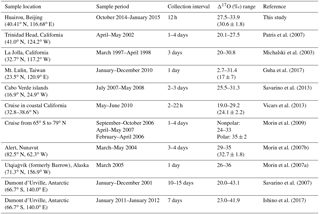${\mathrm{\Delta }}^{\mathrm{17}}\mathrm{O}\left({\mathrm{NO}}_{\mathrm{3}}^{-}\right)$ ranged from 27.5 ‰ to 33.9 ‰ with a mean of (29.1±1.3) ‰ on NPD and (31.0±1.7) ‰ on PD (Fig. 3c). Our observed ${\mathrm{\Delta }}^{\mathrm{17}}\mathrm{O}\left({\mathrm{NO}}_{\mathrm{3}}^{-}\right)$ is in the range of aerosol ${\mathrm{\Delta }}^{\mathrm{17}}\mathrm{O}\left({\mathrm{NO}}_{\mathrm{3}}^{-}\right)$ reported in the literature (Table 3) and wet deposition ${\mathrm{\Delta }}^{\mathrm{17}}\mathrm{O}\left({\mathrm{NO}}_{\mathrm{3}}^{-}\right)$ observed in East Asia (Nelson et al., 2018; Tsunogai et al., 2010, 2016). All our observed ${\mathrm{\Delta }}^{\mathrm{17}}\mathrm{O}\left({\mathrm{NO}}_{\mathrm{3}}^{-}\right)$ values, whether from the daytime sample (08:00–20:00) or the nighttime sample (20:00–08:00), are larger than 25 ‰, the maximum of ${\mathrm{\Delta }}^{\mathrm{17}}\mathrm{O}\left({\mathrm{NO}}_{\mathrm{3}}^{-}\right)$ that can be produced via NO2+OH and NO2+H2O (Table 1) with the assumption of bulk Δ17O(O3)=26 ‰ (Ishino et al., 2017; Vicars and Savarino, 2014). This directly suggests that nocturnal formation pathways (${\mathrm{N}}_{\mathrm{2}}{\mathrm{O}}_{\mathrm{5}}+{\mathrm{H}}_{\mathrm{2}}\mathrm{O}/{\mathrm{Cl}}^{-}$ and NO3+HC) must contribute to all the sampled nitrate. Given that the lifetime of atmospheric nitrate is typically larger than our sampling collection interval (Vicars et al., 2013), each of our samples is expected to reflect both daytime and nocturnal nitrate production. Not surprisingly, the ${\mathrm{\Delta }}^{\mathrm{17}}\mathrm{O}\left({\mathrm{NO}}_{\mathrm{3}}^{-}\right)$ mean of daytime and nighttime samples is (30.3±1.5) ‰ and (30.9±2.1) ‰, respectively, showing no significant difference at the 0.01 level (t test).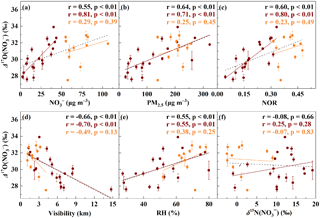Figure 4Relationships between ${\mathrm{\Delta }}^{\mathrm{17}}\mathrm{O}\left({\mathrm{NO}}_{\mathrm{3}}^{-}\right)$ and other parameters. The relationship between ${\mathrm{\Delta }}^{\mathrm{17}}\mathrm{O}\left({\mathrm{NO}}_{\mathrm{3}}^{-}\right)$ and ${\mathrm{NO}}_{\mathrm{3}}^{-}$concentrations (a), PM2.5 concentrations (b), nitrogen oxidation ratio (NOR, c), visibility (d), relative humidity (RH, e), and ${\mathit{\delta }}^{\mathrm{15}}\mathrm{N}\left({\mathrm{NO}}_{\mathrm{3}}^{-}\right)$ (f). The dark red dots are samples with ${\mathrm{NO}}_{\mathrm{3}}^{-}$< 50 µg m−3 and the orange dots are samples with ${\mathrm{NO}}_{\mathrm{3}}^{-}$> 50 µg m−3. The black dashed lines are linear least-squares fitting lines for all samples, the dark red solid lines are linear least-squares fitting lines for samples with ${\mathrm{NO}}_{\mathrm{3}}^{-}$< 50 µg m−3, and the orange solid lines are linear least-squares fitting lines for samples with ${\mathrm{NO}}_{\mathrm{3}}^{-}$> 50 µg m−3. The error bars are ±1σ of replicate measurements of each sample.

${\mathit{\delta }}^{\mathrm{15}}\mathrm{N}\left({\mathrm{NO}}_{\mathrm{3}}^{-}\right)$ in our observation varied from −2.5 ‰ to 19.2 ‰ with a mean of (7.4±6.8) ‰, which is in the range of ${\mathit{\delta }}^{\mathrm{15}}\mathrm{N}\left({\mathrm{NO}}_{\mathrm{3}}^{-}\right)$ observed from rainwater in Beijing, China (Zhang et al., 2008), and similar to ${\mathit{\delta }}^{\mathrm{15}}\mathrm{N}\left({\mathrm{NO}}_{\mathrm{3}}^{-}\right)$ values observed from aerosols in Germany (Freyer, 1991). Figure 3d shows that ${\mathit{\delta }}^{\mathrm{15}}\mathrm{N}\left({\mathrm{NO}}_{\mathrm{3}}^{-}\right)$ varies largely in October 2014. The mean ${\mathit{\delta }}^{\mathrm{15}}\mathrm{N}\left({\mathrm{NO}}_{\mathrm{3}}^{-}\right)$ varied from (0.4±1.5) ‰ in the period 08:00 on 18 October–08:00 on 21 October to (10.7±1.4) ‰ in the period 08:00 on 21 October–08:00 on 23 October and then decreased to ($-\mathrm{0.9}±\mathrm{2.1}$) ‰ in the period 08:00 on 23 October–08:00 on 26 October, which corresponds to PM2.5 concentrations being 155±63, 57±19, and (188±51) µg m−3 respectively. However, during the residential heating season, relatively high ${\mathit{\delta }}^{\mathrm{15}}\mathrm{N}\left({\mathrm{NO}}_{\mathrm{3}}^{-}\right)$ (7.6 ‰–19.2 ‰) levels were always observed, both on NPD and PD. This may be related to the high NOX emission from coal combustion in north China (Wang et al., 2012; Lin, 2012; Zhang et al., 2007).

## 3.2 Relationships between ${\mathrm{\Delta }}^{\mathrm{17}}\mathrm{O}\left({\mathrm{NO}}_{\mathrm{3}}^{-}\right)$ and other data

Figure 4 presents the relationships between ${\mathrm{\Delta }}^{\mathrm{17}}\mathrm{O}\left({\mathrm{NO}}_{\mathrm{3}}^{-}\right)$ and ${\mathrm{NO}}_{\mathrm{3}}^{-}$ concentrations, PM2.5 concentrations, NOR, visibility, RH, and ${\mathit{\delta }}^{\mathrm{15}}\mathrm{N}\left({\mathrm{NO}}_{\mathrm{3}}^{-}\right)$. ${\mathrm{\Delta }}^{\mathrm{17}}\mathrm{O}\left({\mathrm{NO}}_{\mathrm{3}}^{-}\right)$ shows a positive correlation with ${\mathrm{NO}}_{\mathrm{3}}^{-}$ concentrations when ${\mathrm{NO}}_{\mathrm{3}}^{-}$< 50 µg m−3 (r=0.81, p<0.01). Similarly, ${\mathrm{\Delta }}^{\mathrm{17}}\mathrm{O}\left({\mathrm{NO}}_{\mathrm{3}}^{-}\right)$ shows a positive correlation with PM2.5 concentration in Fig. 4b and NOR in Fig. 4c when ${\mathrm{NO}}_{\mathrm{3}}^{-}$< 50 µg m−3 (r=0.71 and r=0.80, p<0.01, respectively). Figure 4d shows that ${\mathrm{\Delta }}^{\mathrm{17}}\mathrm{O}\left({\mathrm{NO}}_{\mathrm{3}}^{-}\right)$ is negatively correlated with visibility in general ($r=-\mathrm{0.66}$, p<0.01). The significant decrease of visibility will largely reduce surface radiation and thereby OH mixing ratios (G. Zheng et al., 2015), which is unfavorable for nitrate production via the NO2+OH pathway. Since the NO2+OH pathway produces low ${\mathrm{\Delta }}^{\mathrm{17}}\mathrm{O}\left({\mathrm{NO}}_{\mathrm{3}}^{-}\right)$ (Table 1), the decreased importance of the NO2+OH pathway will conversely increase ${\mathrm{\Delta }}^{\mathrm{17}}\mathrm{O}\left({\mathrm{NO}}_{\mathrm{3}}^{-}\right)$. While the rise of RH accompanying the large increase of PM2.5 favors nitrate production via the heterogeneous uptake of gases, e.g., N2O5 (G. Zheng et al., 2015; B. Zheng et al., 2015), and the heterogeneous uptake of N2O5 produces relative high ${\mathrm{\Delta }}^{\mathrm{17}}\mathrm{O}\left({\mathrm{NO}}_{\mathrm{3}}^{-}\right)$ (Table 1), the enhanced heterogeneous uptake of N2O5 will increase ${\mathrm{\Delta }}^{\mathrm{17}}\mathrm{O}\left({\mathrm{NO}}_{\mathrm{3}}^{-}\right)$ too. Therefore, the decrease of the importance of NO2+OH and the increase of the importance of the heterogeneous uptake of N2O5 should be responsible for the positive correlation between ${\mathrm{\Delta }}^{\mathrm{17}}\mathrm{O}\left({\mathrm{NO}}_{\mathrm{3}}^{-}\right)$ and ${\mathrm{NO}}_{\mathrm{3}}^{-}$ concentrations. In addition, for samples with ${\mathrm{NO}}_{\mathrm{3}}^{-}$> 50 µg m−3, visibility was always low with narrow variations (2.3±1.0 km), and RH was always high with a narrow range (67±7 %), which may be the reason for the relatively high ${\mathrm{\Delta }}^{\mathrm{17}}\mathrm{O}\left({\mathrm{NO}}_{\mathrm{3}}^{-}\right)$ being observed (31.2±1.7 ‰). Figure 4f shows that ${\mathrm{\Delta }}^{\mathrm{17}}\mathrm{O}\left({\mathrm{NO}}_{\mathrm{3}}^{-}\right)$ is not correlated with ${\mathit{\delta }}^{\mathrm{15}}\mathrm{N}\left({\mathrm{NO}}_{\mathrm{3}}^{-}\right)$.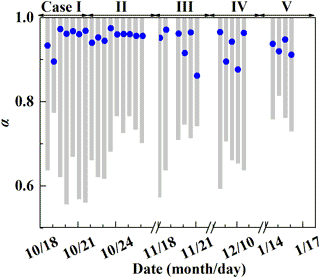Figure 5Estimate of the proportion of O3 oxidation in NOX cycling, α. The gray column represents the possible α range determined by ${\mathrm{\Delta }}^{\mathrm{17}}\mathrm{O}\left({\mathrm{NO}}_{\mathrm{3}}^{-}\right)$. The blue dot represents a specific α value calculated by Eq. (3).

Table 4The possible range of fractional contribution of different nitrate formation pathways during PD of each case estimated on the basis of observed ${\mathrm{\Delta }}^{\mathrm{17}}\mathrm{O}\left({\mathrm{NO}}_{\mathrm{3}}^{-}\right)$a.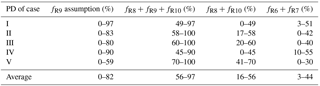a R6, R7, R8, R9, and R10 are, respectively, NO2+OH, NO2+H2O, NO3+HC, N2O5+H2O, and ${\mathrm{N}}_{\mathrm{2}}{\mathrm{O}}_{\mathrm{5}}+{\mathrm{Cl}}^{-}$ pathways.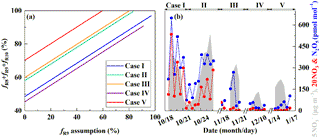Figure 6Estimate of the nocturnal formation pathways. The estimated relative importance of nocturnal formation pathways (${f}_{\mathrm{R}\mathrm{8}}+{f}_{\mathrm{R}\mathrm{9}}+{f}_{\mathrm{R}\mathrm{10}}\right)$ during PD of each case on the basis of observed ${\mathrm{\Delta }}^{\mathrm{17}}\mathrm{O}\left({\mathrm{NO}}_{\mathrm{3}}^{-}\right)$ (see Sect. 2.3, a) and the simulated mixing ratios of N2O5 and NO3 radicals by MCM (b). R8, R9, and R10 in (a) represent NO3+HC, N2O5+H2O, and ${\mathrm{N}}_{\mathrm{2}}{\mathrm{O}}_{\mathrm{5}}+{\mathrm{Cl}}^{-}$ pathways, respectively.

## 3.3 Estimate of nocturnal formation pathways

Before estimating the relative importance of different nitrate formation pathways, we estimate the proportion of O3 oxidation in the NO2 production rate, α. The possible α range can be calculated based on observed ${\mathrm{\Delta }}^{\mathrm{17}}\mathrm{O}\left({\mathrm{NO}}_{\mathrm{3}}^{-}\right)$. It can be obtained from Table 1 that 25α ‰ <${\mathrm{\Delta }}^{\mathrm{17}}\mathrm{O}\left({\mathrm{NO}}_{\mathrm{3}}^{-}\right)$< (25α+14) ‰, so the lower limit of possible α is (${\mathrm{\Delta }}^{\mathrm{17}}\mathrm{O}\left({\mathrm{NO}}_{\mathrm{3}}^{-}\right)$−14 ‰) ∕25 ‰. And since ${\mathrm{\Delta }}^{\mathrm{17}}\mathrm{O}\left({\mathrm{NO}}_{\mathrm{3}}^{-}\right)\ge \mathrm{27.5}$ ‰ in our observation, the higher limit of α is always 1 for all the samples. Figure 5 presents the possible range of calculated α based on ${\mathrm{\Delta }}^{\mathrm{17}}\mathrm{O}\left({\mathrm{NO}}_{\mathrm{3}}^{-}\right)$. The calculated lower limit of α ranged from 0.56 to 0.81 with a mean of 0.68±0.07, which directly suggests that O3 oxidation played a dominated role in NOX cycling during Beijing haze. To estimate the specific α value, chemical kinetics in Table 2 and Eq. (3) were used. Specific α is estimated to range from 0.86 to 0.97 with a mean of (0.94±0.03), which is in the possible range of α value calculated directly based on ${\mathrm{\Delta }}^{\mathrm{17}}\mathrm{O}\left({\mathrm{NO}}_{\mathrm{3}}^{-}\right)$ (Fig. 5) and close to the range of 0.85–1 determined in other midlatitude areas (Michalski et al., 2003; Patris et al., 2007).

Figure 6a shows the estimated relative importance of nocturnal formation pathways (${\mathrm{N}}_{\mathrm{2}}{\mathrm{O}}_{\mathrm{5}}+{\mathrm{H}}_{\mathrm{2}}\mathrm{O}/{\mathrm{Cl}}^{-}$ and NO3+HC) during PD of each case on the basis of observed ${\mathrm{\Delta }}^{\mathrm{17}}\mathrm{O}\left({\mathrm{NO}}_{\mathrm{3}}^{-}\right)$. Possible fractional contributions of nocturnal formation pathways range from 49 to 97 %, 58 to 100 %, 60 to 100 %, 45–90 % and 70–100 % on PD of Case I to V, respectively, with a mean of 56–97 %. This directly implies that nocturnal chemistry dominates atmospheric nitrate production in Beijing haze. This finding is consistent with the suggested importance of the heterogeneous uptake of N2O5 during Beijing haze by previous studies (Su et al., 2017; Wang et al., 2017b). The other pathways (NO2+OH and NO2+H2O) account for the remaining fraction with a mean possible range of 3–44 %. Since NO2+OH and NO2+H2O produce the same ${\mathrm{\Delta }}^{\mathrm{17}}\mathrm{O}\left({\mathrm{NO}}_{\mathrm{3}}^{-}\right)$ signature in our assumptions (Table 1), we cannot distinguish their fractional contributions purely from the observed ${\mathrm{\Delta }}^{\mathrm{17}}\mathrm{O}\left({\mathrm{NO}}_{\mathrm{3}}^{-}\right)$ in the present study. However, the overall positive correlation between ${\mathrm{\Delta }}^{\mathrm{17}}\mathrm{O}\left({\mathrm{NO}}_{\mathrm{3}}^{-}\right)$ and RH (r=0.55, p<0.01; Fig. 4e) suggests that the heterogeneous uptake of NO2 should be less important than the heterogeneous uptake of N2O5; otherwise, a negative relationship between ${\mathrm{\Delta }}^{\mathrm{17}}\mathrm{O}\left({\mathrm{NO}}_{\mathrm{3}}^{-}\right)$ and RH is expected. Our calculations also suggest that the sum of possible fractional contributions of ${\mathrm{N}}_{\mathrm{2}}{\mathrm{O}}_{\mathrm{5}}+{\mathrm{Cl}}^{-}$ and NO3+HC is in the range of 0–49 %, 17–58 %, 20–60 %, 0–45 %, and 41–70 % on PD of Case I to V, respectively, with a mean of 16–56 % (Table 4), which emphasizes that ${\mathrm{N}}_{\mathrm{2}}{\mathrm{O}}_{\mathrm{5}}+{\mathrm{Cl}}^{-}$ and NO3+HC played a unignorable role in nitrate production during Beijing haze. Due to the fact that ${\mathrm{N}}_{\mathrm{2}}{\mathrm{O}}_{\mathrm{5}}+{\mathrm{Cl}}^{-}$ and NO3+HC produce the same ${\mathrm{\Delta }}^{\mathrm{17}}\mathrm{O}\left({\mathrm{NO}}_{\mathrm{3}}^{-}\right)$ in our assumptions (Table 1), we cannot distinguish their fractional contributions purely from the observed ${\mathrm{\Delta }}^{\mathrm{17}}\mathrm{O}\left({\mathrm{NO}}_{\mathrm{3}}^{-}\right)$ in this study either. However, NO3+HC should be minor for nitrate production. For example, the 3-D modeling work of Alexander et al. (2009) suggests that the NO3+HC pathway only accounts for 4 % of global tropospheric nitrate production annually on average, and Michalski et al. (2003) found that the NO3+HC pathway contributes 1–10 % to nitrate production on the basis of an annual observation at La Jolla, California, with low values in winter. Therefore, in addition to NO3+HC, ${\mathrm{N}}_{\mathrm{2}}{\mathrm{O}}_{\mathrm{5}}+{\mathrm{Cl}}^{-}$ is likely to also contribute to nitrate production during haze in Beijing. In support of this, the concentrations of Cl are as high as (5.5±4.1) µg m−3 during PD of all the cases in our observation and the mixing ratios of ClNO2, an indicator of the ${\mathrm{N}}_{\mathrm{2}}{\mathrm{O}}_{\mathrm{5}}+{\mathrm{Cl}}^{-}$ pathway, reached up to 2.9 nmol mol−1 during a summer observation in suburban Beijing (Wang et al., 2018b) and reached up to 5.0 nmol mol−1 in another modeling work in summer rural Beijing (Wang et al., 2017c).

Figure 6b presents the simulated mixing ratios of surface N2O5 and NO3 radicals during our observational period by using the box model MCM. The 12 h averaged mixing ratios of simulated N2O5 ranged from 3 to 649 pmol mol−1, while simulated NO3 radicals ranged from 0 to 27 pmol mol−1. In comparison, previous observations in Beijing suggest that 5 s averaged N2O5 can be as high as 1.3 nmol mol−1 and 30 min averaged NO3 radicals can be as high as 38 pmol mol−1, with large day-to-day variability (Wang et al., 2015, 2017b). During Case I and II in October, simulated N2O5 and NO3 radicals present similar trends with the observed ${\mathrm{NO}}_{\mathrm{3}}^{-}$ and remain relatively high during PD (346±128 and 9±7 pmol mol−1, respectively, Fig. 6b), which supports the dominant role of nocturnal formation pathways suggested by ${\mathrm{\Delta }}^{\mathrm{17}}\mathrm{O}\left({\mathrm{NO}}_{\mathrm{3}}^{-}\right)$. However, during Case III–V in the residential heating season, the simulated surface mixing ratios of N2O5 and NO3 radicals remain relatively low during PD (63±80 and <1 pmol mol−1, respectively, Fig. 6b), which seems to be inconsistent with ${\mathrm{\Delta }}^{\mathrm{17}}\mathrm{O}\left({\mathrm{NO}}_{\mathrm{3}}^{-}\right)$ observations. We note that a recent study suggests that the heterogeneous uptake of N2O5 is negligible at the surface but larger at higher altitudes (e.g., >150 m) during winter haze in Beijing (Wang et al., 2018a). So during PD of Case III–V in our observational period, large nitrate production via heterogeneous uptake of N2O5 may occur aloft rather than at the surface, which leads to the dominant role of nocturnal formation pathways as suggested by ${\mathrm{\Delta }}^{\mathrm{17}}\mathrm{O}\left({\mathrm{NO}}_{\mathrm{3}}^{-}\right)$.

4 Conclusions

We report the first observation of isotopic composition (Δ17O and δ15N) of atmospheric nitrate in Beijing haze. The observed ${\mathrm{\Delta }}^{\mathrm{17}}\mathrm{O}\left({\mathrm{NO}}_{\mathrm{3}}^{-}\right)$ ranged from 27.5 ‰ to 33.9 ‰ with a mean of (30.6±1.8) ‰. ${\mathit{\delta }}^{\mathrm{15}}\mathrm{N}\left({\mathrm{NO}}_{\mathrm{3}}^{-}\right)$ ranged largely from −2.5 ‰ to 19.2 ‰ with a mean of (7.4±6.8) ‰. When ${\mathrm{NO}}_{\mathrm{3}}^{-}$ is <50µg m−3, a positive correlation was observed between ${\mathrm{\Delta }}^{\mathrm{17}}\mathrm{O}\left({\mathrm{NO}}_{\mathrm{3}}^{-}\right)$ and ${\mathrm{NO}}_{\mathrm{3}}^{-}$ concentration (r=0.81, p<0.01). This is likely to result from the variation of relative importance of different nitrate formation pathways. Calculations with the constraint of ${\mathrm{\Delta }}^{\mathrm{17}}\mathrm{O}\left({\mathrm{NO}}_{\mathrm{3}}^{-}\right)$ suggest that nocturnal pathways (${\mathrm{N}}_{\mathrm{2}}{\mathrm{O}}_{\mathrm{5}}+{\mathrm{H}}_{\mathrm{2}}\mathrm{O}/{\mathrm{Cl}}^{-}$ and NO3+HC) dominated nitrate production during polluted days (PM2.5≥75µg m−3), with a mean possible contribution of 56–97 %. ${\mathrm{\Delta }}^{\mathrm{17}}\mathrm{O}\left({\mathrm{NO}}_{\mathrm{3}}^{-}\right)$ also indicates that O3 dominated NO oxidation during Beijing haze.

Data availability

All data needed to draw the conclusions are present in the main text and/or the Supplement. For additional data, please contact the corresponding author (zqxie@ustc.edu.cn).

Supplement

Author contributions

ZX conceived this study. PH conducted isotope measurements. PH, XC, SF, HZ, and HK performed the field experiments and ion measurements. PH, ZX, and XY interpreted the data. CL contributed to the field observation support. PH wrote the manuscript with input from ZX. All authors were involved in the discussion and revision of the manuscript.

Competing interests

The authors declare that they have no conflict of interest.

Special issue statement

Acknowledgements

This work was supported by the National Key Project of MOST (2016YFC0203302), NSFC (91544013), the Key Project of CAS (KJZD-EW-TZ-G06-01), and the Atmospheric Pollution Control of the Prime Minister (DQGG0104). We gratefully thank staff of IsoLab at UW for their technical support, and Becky Alexander and Lei Geng for helpful discussions.

Edited by: Jian Wang
Reviewed by: Jochen Rudolph, Greg Michalski, and two anonymous referees

References

Alexander, B., Hastings, M. G., Allman, D. J., Dachs, J., Thornton, J. A., and Kunasek, S. A.: Quantifying atmospheric nitrate formation pathways based on a global model of the oxygen isotopic composition (Δ17O) of atmospheric nitrate, Atmos. Chem. Phys., 9, 5043–5056, https://doi.org/10.5194/acp-9-5043-2009, 2009.

Berhanu, T. A., Savarino, J., Bhattacharya, S. K., and Vicars, W. C.: 17O excess transfer during the ${\mathrm{NO}}_{\mathrm{2}}+{\mathrm{O}}_{\mathrm{3}}\to {\mathrm{NO}}_{\mathrm{3}}+{\mathrm{O}}_{\mathrm{2}}$ reaction, J. Chem. Phys., 136, 044311, https://doi.org/10.1063/1.3666852, 2012.

Bertram, T. H. and Thornton, J. A.: Toward a general parameterization of N2O5 reactivity on aqueous particles: the competing effects of particle liquid water, nitrate and chloride, Atmos. Chem. Phys., 9, 8351–8363, https://doi.org/10.5194/acp-9-8351-2009, 2009.

Beyn, F., Matthias, V., and Dähnke, K.: Changes in atmospheric nitrate deposition in Germany – An isotopic perspective, Environ. Pollut., 194, 1–10, 2014.

Brenninkmeijer, C. A., Janssen, C., Kaiser, J., Röckmann, T., Rhee, T. S., and Assonov, S. S.: Isotope effects in the chemistry of atmospheric trace compounds, Chem. Rev., 103, 5125–5162, 2003.

Brook, R. D., Rajagopalan, S., Pope, C. A., Brook, J. R., Bhatnagar, A., Diez-Roux, A. V., Holguin, F., Hong, Y., Luepker, R. V., and Mittleman, M. A.: Particulate matter air pollution and cardiovascular disease an update to the scientific statement from the American Heart Association, Circulation, 121, 2331–2378, 2010.

Brown, S. S. and Stutz, J.: Nighttime radical observations and chemistry, Chem. Soc. Rev., 41, 6405–6447, 2012.

Burkholder, J. B., Sander, S. P., Abbatt, J. P. D., Barker, J. R., Huie, R. E., Kolb, C. E., Kurylo, M. J., Orkin, V. L., Wilmouth, D. M., and Wine, P. H.: Chemical Kinetics and Photochemical Data for Use in Atmospheric Studies: Evaluation Number 18, Pasadena, CA, Jet Propulsion Laboratory, National Aeronautics and Space Administration, 2015.

Chen, Q., Geng, L., Schmidt, J. A., Xie, Z., Kang, H., Dachs, J., Cole-Dai, J., Schauer, A. J., Camp, M. G., and Alexander, B.: Isotopic constraints on the role of hypohalous acids in sulfate aerosol formation in the remote marine boundary layer, Atmos. Chem. Phys., 16, 11433–11450, https://doi.org/10.5194/acp-16-11433-2016, 2016.

Chen, Z., Zhang, J., Zhang, T., Liu, W., and Liu, J.: Haze observations by simultaneous lidar and WPS in Beijing before and during APEC, 2014, Sci. China Chem., 58, 1385–1392, 2015.

Cheng, Z., Jiang, J., Fajardo, O., Wang, S., and Hao, J.: Characteristics and health impacts of particulate matter pollution in China (2001–2011), Atmos. Environ., 65, 186–194, 2013.

Cheung, J. L., Li, Y., Boniface, J., Shi, Q., Davidovits, P., Worsnop, D. R., Jayne, J. T., and Kolb, C. E.: Heterogeneous interactions of NO2 with aqueous surfaces, J. Phys. Chem. A, 104, 2655–2662, 2000.

Elliott, E. M., Kendall, C., Boyer, E. W., Burns, D. A., Lear, G. G., Golden, H. E., Harlin, K., Bytnerowicz, A., Butler, T. J., and Glatz, R.: Dual nitrate isotopes in dry deposition: Utility for partitioning NOx source contributions to landscape nitrogen deposition, J. Geophys. Res.-Biogeo., 114, G04020, https://doi.org/10.1029/2008JG000889, 2009.

Elshorbany, Y. F., Kleffmann, J., Hofzumahaus, A., Kurtenbach, R., Wiesen, P., Brauers, T., Bohn, B., Dorn, H. P., Fuchs, H., and Holland, F.: HOx budgets during HOxComp: A case study of HOx chemistry under NOx-limited conditions, J. Geophys. Res., 117, D03307, https://doi.org/10.1029/2011JD017008, 2012.

Fang, Y. T., Koba, K., Wang, X. M., Wen, D. Z., Li, J., Takebayashi, Y., Liu, X. Y., and Yoh, M.: Anthropogenic imprints on nitrogen and oxygen isotopic composition of precipitation nitrate in a nitrogen-polluted city in southern China, Atmos. Chem. Phys., 11, 1313–1325, https://doi.org/10.5194/acp-11-1313-2011, 2011.

Felix, J. D., Elliott, E. M., and Shaw, S. L.: Nitrogen isotopic composition of coal-fired power plant NOx: influence of emission controls and implications for global emission inventories, Environ. Sci. Technol., 46, 3528–3535, 2012.

Freyer, H. D.: Seasonal variation of 15N∕14N ratios in atmospheric nitrate species, Tellus B, 43, 30–44, 1991.

Freyer, H. D., Kley, D., Volz-Thomas, A., and Kobel, K.: On the interaction of isotopic exchange processes with photochemical reactions in atmospheric oxides of nitrogen, J. Geophys. Res.-Atmos., 98, 14791–14796, 1993.

Geng, L., Alexander, B., Cole-Dai, J., Steig, E. J., Savarino, J., Sofen, E. D., and Schauer, A. J.: Nitrogen isotopes in ice core nitrate linked to anthropogenic atmospheric acidity change, P. Natl. Acad. Sci. USA, 111, 5808–5812, 2014.

Goodman, A. L., Underwood, G. M., and Grassian, V. H.: Heterogeneous reaction of NO2: Characterization of gas-phase and adsorbed products from the reaction, 2NO2(g) +H2O(a) HONO(g) +HNO3(a) on hydrated silica particles, J. Phys. Chem. A, 103, 7217–7223, 1999.

Guha, T., Lin, C. T., Bhattacharya, S. K., Mahajan, A. S., Ou-Yang, C.-F., Lan, Y.-P., Hsu, S. C., and Liang, M.-C.: Isotopic ratios of nitrate in aerosol samples from Mt. Lulin, a high-altitude station in Central Taiwan, Atmos. Environ., 154, 53–69, 2017.

Hastings, M. G., Casciotti, K. L., and Elliott, E. M.: Stable isotopes as tracers of anthropogenic nitrogen sources, deposition, and impacts, Elements, 9, 339–344, 2013.

He, P., Alexander, B., Geng, L., Chi, X., Fan, S., Zhan, H., Kang, H., Zheng, G., Cheng, Y., Su, H., Liu, C., and Xie, Z.: Isotopic constraints on heterogeneous sulfate production in Beijing haze, Atmos. Chem. Phys., 18, 5515–5528, https://doi.org/10.5194/acp-18-5515-2018, 2018.

Hoering, T.: The isotopic composition of the ammonia and the nitrate ion in rain, Geochim. Cosmochim. Acta, 12, 97–102, 1957.

Ishino, S., Hattori, S., Savarino, J., Jourdain, B., Preunkert, S., Legrand, M., Caillon, N., Barbero, A., Kuribayashi, K., and Yoshida, N.: Seasonal variations of triple oxygen isotopic compositions of atmospheric sulfate, nitrate, and ozone at Dumont d'Urville, coastal Antarctica, Atmos. Chem. Phys., 17, 3713–3727, https://doi.org/10.5194/acp-17-3713-2017, 2017.

Kaiser, J., Hastings, M. G., Houlton, B. Z., Röckmann, T., and Sigman, D. M.: Triple oxygen isotope analysis of nitrate using the denitrifier method and thermal decomposition of N2O, Anal. Chem., 79, 599–607, 2007.

Kanaya, Y., Cao, R., Akimoto, H., Fukuda, M., Komazaki, Y., Yokouchi, Y., Koike, M., Tanimoto, H., Takegawa, N., and Kondo, Y.: Urban photochemistry in central Tokyo: 1. Observed and modeled OH and HO2 radical concentrations during the winter and summer of 2004, J. Geophys. Res., 112, D21312, https://doi.org/10.1029/2007JD008670, 2007.

Kunasek, S. A., Alexander, B., Steig, E. J., Hastings, M. G., Gleason, D. J., and Jarvis, J. C.: Measurements and modeling of Δ17O of nitrate in snowpits from Summit, Greenland, J. Geophys. Res., 113, D24302, https://doi.org/10.1029/2008JD010103, 2008.

Li, H. J., Zhu, T., Zhao, D. F., Zhang, Z. F., and Chen, Z. M.: Kinetics and mechanisms of heterogeneous reaction of NO2 on CaCO3 surfaces under dry and wet conditions, Atmos. Chem. Phys., 10, 463–474, https://doi.org/10.5194/acp-10-463-2010, 2010.

Li, Z., Hu, R., Xie, P., Wang, H., Lu, K., and Wang, D.: Intercomparison of in situ CRDS and CEAS for measurements of atmospheric N2O5 in Beijing, China, Sci. Total Environ., 613, 131–139, 2018.

Liang, J., Horowitz, L. W., Jacob, D. J., Wang, Y., Fiore, A. M., Logan, J. A., Gardner, G. M., and Munger, J. W.: Seasonal budgets of reactive nitrogen species and ozone over the United States, and export fluxes to the global atmosphere, J. Geophys. Res.-Atmos., 103, 13435–13450, 1998.

Lin, J.-T.: Satellite constraint for emissions of nitrogen oxides from anthropogenic, lightning and soil sources over East China on a high-resolution grid, Atmos. Chem. Phys., 12, 2881–2898, https://doi.org/10.5194/acp-12-2881-2012, 2012.

Lin, W., Xu, X., Ge, B., and Liu, X.: Gaseous pollutants in Beijing urban area during the heating period 2007–2008: variability, sources, meteorological, and chemical impacts, Atmos. Chem. Phys., 11, 8157–8170, https://doi.org/10.5194/acp-11-8157-2011, 2011.

Liu, Z., Wang, Y., Gu, D., Zhao, C., Huey, L. G., Stickel, R., Liao, J., Shao, M., Zhu, T., Zeng, L., Amoroso, A., Costabile, F., Chang, C.-C., and Liu, S.-C.: Summertime photochemistry during CAREBeijing-2007: ROx budgets and O3 formation, Atmos. Chem. Phys., 12, 7737–7752, https://doi.org/10.5194/acp-12-7737-2012, 2012.

Michalski, G., Scott, Z., Kabiling, M., and Thiemens, M. H.: First measurements and modeling of Δ17O in atmospheric nitrate, Geophys. Res. Lett., 30, 1870, https://doi.org/10.1029/2003GL017015, 2003.

Mihelcic, D., Holland, F., Hofzumahaus, A., Hoppe, L., Konrad, S., Müsgen, P., Pätz, H. W., Schäfer, H. J., Schmitz, T., and Volz-Thomas, A.: Peroxy radicals during BERLIOZ at Pabstthum: Measurements, radical budgets and ozone production, J. Geophys. Res., 108, 8254, https://doi.org/10.1029/2001JD001014, 2003.

Morin, S., Savarino, J., Bekki, S., Cavender, A., Shepson, P. B., and Bottenheim, J. W.: Major influence of BrO on the NOx and nitrate budgets in the Arctic spring, inferred from ${\mathrm{\Delta }}^{\mathrm{17}}\mathrm{O}\left({\mathrm{NO}}_{\mathrm{3}}^{-}\right)$ measurements during ozone depletion events, Environ. Chem., 4, 238–241, https://doi.org/10.1071/EN07003, 2007a.

Morin, S., Savarino, J., Bekki, S., Gong, S., and Bottenheim, J. W.: Signature of Arctic surface ozone depletion events in the isotope anomaly (Δ17O) of atmospheric nitrate, Atmos. Chem. Phys., 7, 1451–1469, https://doi.org/10.5194/acp-7-1451-2007, 2007b.

Morin, S., Savarino, J., Frey, M. M., Yan, N., Bekki, S., Bottenheim, J. W., and Martins, J. M.: Tracing the origin and fate of NOx in the Arctic atmosphere using stable isotopes in nitrate, Science, 322, 730–732, 2008.

Morin, S., Savarino, J., Frey, M. M., Domine, F., Jacobi, H. W., Kaleschke, L., and Martins, J. M.: Comprehensive isotopic composition of atmospheric nitrate in the Atlantic Ocean boundary layer from 65 S to 79 N, J. Geophys. Res.-Atmos., 114, D05303, https://doi.org/10.1029/2008JD010696, 2009.

Morin, S., Sander, R., and Savarino, J.: Simulation of the diurnal variations of the oxygen isotope anomaly (Δ17O) of reactive atmospheric species, Atmos. Chem. Phys., 11, 3653–3671, https://doi.org/10.5194/acp-11-3653-2011, 2011.

Nelson, D. M., Tsunogai, U., Ding, D., Ohyama, T., Komatsu, D. D., Nakagawa, F., Noguchi, I., and Yamaguchi, T.: Triple oxygen isotopes indicate urbanization affects sources of nitrate in wet and dry atmospheric deposition, Atmos. Chem. Phys., 18, 6381–6392, https://doi.org/10.5194/acp-18-6381-2018, 2018.

Pathak, R. K., Wu, W. S., and Wang, T.: Summertime PM2.5 ionic species in four major cities of China: nitrate formation in an ammonia-deficient atmosphere, Atmos. Chem. Phys., 9, 1711–1722, https://doi.org/10.5194/acp-9-1711-2009, 2009.

Pathak, R. K., Wang, T., and Wu, W. S.: Nighttime enhancement of PM2.5 nitrate in ammonia-poor atmospheric conditions in Beijing and Shanghai: plausible contributions of heterogeneous hydrolysis of N2O5 and HNO3 partitioning, Atmos. Environ., 45, 1183–1191, 2011.

Patris, N., Cliff, S. S., Quinn, P. K., Kasem, M., and Thiemens, M. H.: Isotopic analysis of aerosol sulfate and nitrate during ITCT-2k2: Determination of different formation pathways as a function of particle size, J. Geophys. Res.-Atmos., 112, D23301, https://doi.org/10.1029/2005JD006214, 2007.

Rao, Z., Chen, Z., Liang, H., Huang, L., and Huang, D.: Carbonyl compounds over urban Beijing: Concentrations on haze and non-haze days and effects on radical chemistry, Atmos. Environ., 124, 207–216, 2016.

Savarino, J. and Thiemens, M. H.: Analytical procedure to determine both δ18O and δ17O of H2O2 in natural water and first measurements, Atmos. Environ., 33, 3683–3690, 1999.

Savarino, J., Kaiser, J., Morin, S., Sigman, D. M., and Thiemens, M. H.: Nitrogen and oxygen isotopic constraints on the origin of atmospheric nitrate in coastal Antarctica, Atmos. Chem. Phys., 7, 1925–1945, https://doi.org/10.5194/acp-7-1925-2007, 2007.

Savarino, J., Bhattacharya, S. K., Morin, S., Baroni, M., and Doussin, J.-F.: The NO +O3 reaction: A triple oxygen isotope perspective on the reaction dynamics and atmospheric implications for the transfer of the ozone isotope anomaly, J. Chem. Phys., 128, 194303, https://doi.org/10.1063/1.2917581, 2008.

Savarino, J., Morin, S., Erbland, J., Grannec, F., Patey, M. D., Vicars, W., Alexander, B., and Achterberg, E. P.: Isotopic composition of atmospheric nitrate in a tropical marine boundary layer, P. Natl. Acad. Sci. USA, 110, 17668–17673, 2013.

Sofen, E. D., Alexander, B., Steig, E. J., Thiemens, M. H., Kunasek, S. A., Amos, H. M., Schauer, A. J., Hastings, M. G., Bautista, J., Jackson, T. L., Vogel, L. E., McConnell, J. R., Pasteris, D. R., and Saltzman, E. S.: WAIS Divide ice core suggests sustained changes in the atmospheric formation pathways of sulfate and nitrate since the 19th century in the extratropical Southern Hemisphere, Atmos. Chem. Phys., 14, 5749–5769, https://doi.org/10.5194/acp-14-5749-2014, 2014.

Su, X., Tie, X., Li, G., Cao, J., Huang, R., Feng, T., Long, X., and Xu, R.: Effect of hydrolysis of N2O5 on nitrate and ammonium formation in Beijing China: WRF-Chem model simulation, Sci. Total Environ., 579, 221–229, 2017.

Sun, Y., Zhuang, G., Tang, A., Wang, Y., and An, Z.: Chemical characteristics of PM2.5 and PM10 in haze-fog episodes in Beijing, Environ. Sci. Technol., 40, 3148–3155, 2006.

Tong, S., Hou, S., Zhang, Y., Chu, B., Liu, Y., He, H., Zhao, P., and Ge, M.: Comparisons of measured nitrous acid (HONO) concentrations in a pollution period at urban and suburban Beijing, in autumn of 2014, Sci. China Chem., 58, 1393–1402, 2015.

Tsunogai, U., Komatsu, D. D., Daita, S., Kazemi, G. A., Nakagawa, F., Noguchi, I., and Zhang, J.: Tracing the fate of atmospheric nitrate deposited onto a forest ecosystem in Eastern Asia using Δ17O, Atmos. Chem. Phys., 10, 1809–1820, https://doi.org/10.5194/acp-10-1809-2010, 2010.

Tsunogai, U., Miyauchi, T., Ohyama, T., Komatsu, D. D., Nakagawa, F., Obata, Y., Sato, K., and Ohizumi, T.: Accurate and precise quantification of atmospheric nitrate in streams draining land of various uses by using triple oxygen isotopes as tracers, Biogeosciences, 13, 3441–3459, https://doi.org/10.5194/bg-13-3441-2016, 2016.

Vicars, W. C., Morin, S., Savarino, J., Wagner, N. L., Erbland, J., Vince, E., Martins, J. M. F., Lerner, B. M., Quinn, P. K., and Coffman, D. J.: Spatial and diurnal variability in reactive nitrogen oxide chemistry as reflected in the isotopic composition of atmospheric nitrate: Results from the CalNex 2010 field study, J. Geophys. Res.-Atmos., 118, 10567–10588, https://doi.org/10.1002/jgrd.50680, 2013.

Vicars, W. C. and Savarino, J.: Quantitative constraints on the 17O-excess (Δ17O) signature of surface ozone: Ambient measurements from 50 N to 50 S using the nitrite-coated filter technique, Geochim. Cosmochim. Acta, 135, 270–287, 2014.

Walters, W. W., Simonini, D. S., and Michalski, G.: Nitrogen isotope exchange between NO and NO2 and its implications for δ15N variations in tropospheric NOx and atmospheric nitrate, Geophys. Res. Lett., 43, 440–448, 2016.

Wang, D., Hu, R., Xie, P., Liu, J., Liu, W., Qin, M., Ling, L., Zeng, Y., Chen, H., Xing, X., Zhu, G., Wu, J., Duan, J., Lu, X., and Shen, L.: Diode laser cavity ring-down spectroscopy for in situ measurement of NO3 radical in ambient air, J. Quant. Spectrosc. Radiat. Transf., 166, 23–29, 2015.

Wang, H., Chen, J., and Lu, K.: Development of a portable cavity-enhanced absorption spectrometer for the measurement of ambient NO3 and N2O5: experimental setup, lab characterizations, and field applications in a polluted urban environment, Atmos. Meas. Tech., 10, 1465–1479, https://doi.org/10.5194/amt-10-1465-2017, 2017a.

Wang, H., Lu, K., Chen, X., Zhu, Q., Chen, Q., Guo, S., Jiang, M., Li, X., Shang, D., and Tan, Z.: High N2O5 concentrations observed in urban Beijing: Implications of a large nitrate formation pathway, Environ. Sci. Technol. Lett., 4, 416–420, 2017b.

Wang, H., Lu, K., Tan, Z., Sun, K., Li, X., Hu, M., Shao, M., Zeng, L., Zhu, T., and Zhang, Y.: Model simulation of NO3, N2O5 and ClNO2 at a rural site in Beijing during CAREBeijing-2006, Atmos. Res., 196, 97–107, 2017c.

Wang, H., Lu, K., Chen, X., Zhu, Q., Wu, Z., Wu, Y., and Sun, K.: Fast particulate nitrate formation via N2O5 uptake aloft in winter in Beijing, Atmos. Chem. Phys., 18, 10483–10495, https://doi.org/10.5194/acp-18-10483-2018, 2018a.

Wang, H., Lu, K., Guo, S., Wu, Z., Shang, D., Tan, Z., Wang, Y., Le Breton, M., Lou, S., Tang, M., Wu, Y., Zhu, W., Zheng, J., Zeng, L., Hallquist, M., Hu, M., and Zhang, Y.: Efficient N2O5 uptake and NO3 oxidation in the outflow of urban Beijing, Atmos. Chem. Phys., 18, 9705–9721, https://doi.org/10.5194/acp-18-9705-2018, 2018b.

Wang, J., Zhang, X., Guo, J., Wang, Z., and Zhang, M.: Observation of nitrous acid (HONO) in Beijing, China: Seasonal variation, nocturnal formation and daytime budget, Sci. Total Environ., 587, 350–359, 2017.

Wang, S. W., Zhang, Q., Streets, D. G., He, K. B., Martin, R. V., Lamsal, L. N., Chen, D., Lei, Y., and Lu, Z.: Growth in NOx emissions from power plants in China: bottom-up estimates and satellite observations, Atmos. Chem. Phys., 12, 4429–4447, https://doi.org/10.5194/acp-12-4429-2012, 2012.

Wang, Y., Zhou, L., Wang, M., and Zheng, X.: Trends of atmospheric methane in Beijing, Chemosphere, 3, 65–71, 2001.

Wen, L., Chen, J., Yang, L., Wang, X., Xu, C., Sui, X., Yao, L., Zhu, Y., Zhang, J., and Zhu, T.: Enhanced formation of fine particulate nitrate at a rural site on the North China Plain in summer: The important roles of ammonia and ozone, Atmos. Environ., 101, 294–302, 2015.

Wu, R., Li, J., Hao, Y., Li, Y., Zeng, L., and Xie, S.: Evolution process and sources of ambient volatile organic compounds during a severe haze event in Beijing, China, Sci. Total Environ., 560, 62–72, 2016.

Xiao, H., Xie, L., Long, A., Ye, F., Pan, Y., Li, D., Long, Z., Chen, L., Xiao, H., and Liu, C.: Use of isotopic compositions of nitrate in TSP to identify sources and chemistry in South China Sea, Atmos. Environ., 109, 70–78, 2015.

Xu, X., Zhao, W., Zhang, Q., Wang, S., Fang, B., Chen, W., Venables, D. S., Wang, X., Pu, W., Wang, X., Gao, X., and Zhang, W.: Optical properties of atmospheric fine particles near Beijing during the HOPE-J3A campaign, Atmos. Chem. Phys., 16, 6421–6439, https://doi.org/10.5194/acp-16-6421-2016, 2016.

Ye, P., Xie, Z., Yu, J., and Kang, H.: Spatial distribution of methanesulphonic acid in the Arctic aerosol collected during the Chinese Arctic Research Expedition, Atmosphere, 6, 699–712, 2015.

Yu, Z. and Elliott, E. M.: Novel Method for Nitrogen Isotopic Analysis of Soil-Emitted Nitric Oxide, Environ. Sci. Technol., 51, 6268–6278, https://doi.org/10.1021/acs.est.7b00592, 2017.

Zhang, J., Chen, Z., Lu, Y., Gui, H., Liu, J., Liu, W., Wang, J., Yu, T., Cheng, Y., and Chen, Y.: Characteristics of aerosol size distribution and vertical backscattering coefficient profile during 2014 APEC in Beijing, Atmos. Environ., 148, 30–41, 2017.

Zhang, Q., Streets, D. G., He, K., Wang, Y., Richter, A., Burrows, J. P., Uno, I., Jang, C. J., Chen, D., Yao, Z., and Lei, Y.: NOx emission trends for China, 1995–2004: The view from the ground and the view from space, J. Geophys. Res., 112, D22306, https://doi.org/10.1029/2007JD008684, 2007.

Zhang, Y., Liu, X., Fangmeier, A., Goulding, K. T. W., and Zhang, F.: Nitrogen inputs and isotopes in precipitation in the North China Plain, Atmos. Environ., 42, 1436–1448, 2008.

Zheng, B., Zhang, Q., Zhang, Y., He, K. B., Wang, K., Zheng, G. J., Duan, F. K., Ma, Y. L., and Kimoto, T.: Heterogeneous chemistry: a mechanism missing in current models to explain secondary inorganic aerosol formation during the January 2013 haze episode in North China, Atmos. Chem. Phys., 15, 2031–2049, https://doi.org/10.5194/acp-15-2031-2015, 2015.

Zheng, G. J., Duan, F. K., Su, H., Ma, Y. L., Cheng, Y., Zheng, B., Zhang, Q., Huang, T., Kimoto, T., Chang, D., Pöschl, U., Cheng, Y. F., and He, K. B.: Exploring the severe winter haze in Beijing: the impact of synoptic weather, regional transport and heterogeneous reactions, Atmos. Chem. Phys., 15, 2969–2983, https://doi.org/10.5194/acp-15-2969-2015, 2015.